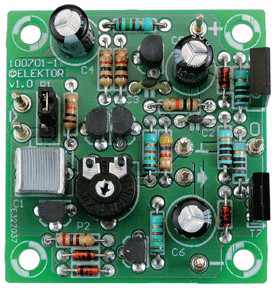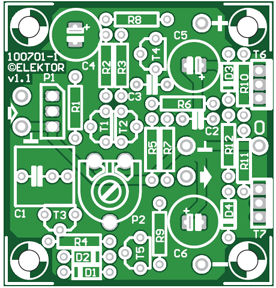# Low-cost Headphone Amplifier

These days it’s not that easy to find a separate headphone amplifier in the shops. They do exist, especially in the hi-fi world, but they come with a matching price tag. The design presented here comes in a bit below these high-end circuits, but can be built using easily obtainable components and still manages to have quite a good sound quality.

## The circuit

The circuit could be described as a type of power-amp, built with discrete components (see Figure 1). At the input we find a volume control (P1, which is connected via a header) and a coupling capacitor (C1), followed by a differential amplifier (Q1, Q2) with a constant current source (Q3) in the emitter branch. The preset between Q1 and Q2 (P2) is used to set the symmetry, or in other words, the output voltage is set to 0 volt DC compared to ground. For the best sound quality we should have the same collector current flowing through both transistors. This can be seen from voltages at test points B and C in the circuit diagram, which are nearly equal. The input offset across R1 is caused by the base current flowing into Q1. This causes the voltage at point A (V(A)) to be slightly negative. A quick measurement of the prototype showed that the base current into Q1 was about 3 μA. Without the offset compensation provided by trimpot P2 the output offset voltage VO would exceed 0.2 V:

VO = (1 + R7/R6) × V(A)
VO = (1 + 10/1.5) × 0.028 = 0.215 VFigure 1. The circuit for the simple headphone amplifier uses easy to get components (one channel shown).

The offset can therefore be removed by setting the differential amplifier to operate slightly asymmetrically. Although this isn’t the best method as far as the sound quality is concerned, it does keep the circuit much simpler.

## Constant current settings

The current source in the emitter branch (Q3) is set to about 3 mA with diodes D1, D2 and resistor R4, which results in Q4 being driven as linearly as possible. The audio signal then makes its way to the driver stage, Q4, which drives the more powerful output transistors (Q6 and Q7). C4 has been added to provide a greater internal gain. The quiescent current in the output stage is set to about 5 mA with Q5 and R9. Assuming a gain (hFE) of 50 in the output transistors, this 5 mA could theoretically provide a linear 0.005 A×50×32 Ω = 8 Vpeak into 32 Ω. However, some limitations are introduced by constant current source Q5 and the voltage drop across the base-emitter junction of Q7 (about 1.5 V). We should also take account of the voltage divider around R11 and R12 (R10 and R12) in the calculations. The maximum voltage Vmax across the load (RL) then becomes

Vmax = RL / (RL + R11 + R12) × (9 – 1.5) Vmax = 4.6 Vpeak

This corresponds to about 3.26 Vrms, which is what we measured, as you can see in the specifications. This means that the circuit can deliver (3.262/32) = 330 mW into 32 Ω, which should be enough to keep most pop and rock fans happy. Resistor R12, which follows the output stage, limits the output current and keeps the circuit stable when a capacitive load is connected, such as a long shielded cable to the headphones. This prevents the output transistors from overheating when there is a short circuit. R10 and R11 keep things symmetric. Despite the value of C2 in the feedback circuit, the bandwidth is still much greater than the audio bandwidth (see the specifications). To obtain a low corner frequency at the input we used 4.7 μF for C1. A capacitor of 2.2 μF (which is easier to obtain) still results in an acceptable corner frequency of 7 Hz (–0.6 dB at 20 Hz). The measurements from one of our prototypes are shown in the circuit diagram. These should be seen as guideline values rather than as exact requirements. The PN junctions and the gain of the transistors can of course vary depending on the manufacturer (this also applies to the current consumption given in the specifications).

## Experimenting

For those of you who don’t mind a little bit more noise (although it will still be inaudible with most headphones), you can increase the impedance of the feedback loop to about 10 kΩ. This can be achieved by increasing R6 and R7 in the parallel circuit to 10 kΩ. In this case the base currents of Q1 and Q2 will compensate each other. If you like experimenting you can replace R6 with a resistor of 12 kΩ and R7 with a resistor of 68 kΩ (perfectionists should use 11.5 kΩ and 76.8 kΩ from the E96 series). It is unlikely that this offers an audible improvement, but there may be a smaller offset this way.

## Construction

A small printed circuit board has been designed for this circuit (see Figure 2). The component layout is shown in Figure 3.Figure 2. The completed circuit is still compact despite the lack of SMDs.Figure 3. The component layout for the no-frills headphone amplifier.

As usual, construction is easiest if you start soldering the lowest components (resistors, diodes) and then continue mounting increasingly higher components (capacitors, transistors, connection pins). You will need two boards for a stereo version, in which case P1 has to be replaced with a stereo potentiometer, so that the volume can be controlled on both channels simultaneously. If your audio source already includes a volume control, you can leave out P1 (put a jumper on the header or solder a wire link on the board from pin 1 and pin 2 of the header instead of the actual header). The input impedance of our suggested circuit (which includes P1) has a minimum of 5 kΩ (P1 set to maximum volume). This shouldn’t be a problem for most modern audio sources. Take note of the pin spacing of decoupling capacitor C1; the board accommodates 5 mm and 7.5 mm versions. For the power supply you could use two 9V batteries. Alternatively, a 2×6 V, 5 VA transformer with a 1.5 A bridge rectifier and 8200 μF/16 V per supply rail is another option. This could optionally be supplemented with a pair of voltage regulators. The output transistors (Q6 and Q7) probably don’t need heatsinks in practice, although a small heatsink will make sure that they will be short circuit proof.

## Component List

#### Resistors

• R1,R7 = 10kΩ
• R2,R3 = 1kΩ
• R4 = 270Ω
• R5 = 4.7kΩ
• R6 = 1.5kΩ
• R8,R9 = 150Ω
• R10,R11,R12 = 10Ω
• P1 = 10kΩ
• P2 = 100Ω trimpot

#### Capacitors

• C1 = 4.7μF, lead pitch 5mm or 7.5mm
• C2 = 6.8pF, lead pitch 5mm
• C3 = 10pF, lead pitch 5mm
• C4,C5,C6 = 100μF 16V radial

#### Semiconductors

• D1,D2,D3,D4 = 1N4148
• Q1,Q2,Q3,Q5 = BC550C
• Q4 = BC560C
• Q6 = BD139
• Q7 = BD140